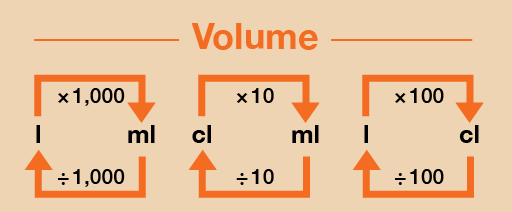Science, Maths & Technology

### Become an OU studentEveryday maths 1

Start this free course now. Just create an account and sign in. Enrol and complete the course for a free statement of participation or digital badge if available.

# 4.1 Changing units

You will sometimes need to change between millilitres and litres. There are 1,000 millilitres in a litre.

Take a look at this section of the metric conversion chart to refer to when you are carrying out the activity below.Figure 34 A conversion chart for volume

## Example: Party food

You are cooking for a large party. The recipe you are using calls for 600 ml of milk to make enough for four people.

How many litres of milk will you need to make ten times as much?

### Method

First you need to multiply the amount in millilitres by 10:

• 600 × 10 = 6,000 ml

However, the question asks for an amount in litres, not millilitres. To convert from millilitres to litres, you need to divide the figure in millilitres by 1,000. So the amount of milk you need in litres is:

• 6,000 ÷ 1,000 = 6 litres

## Activity 10: Converting between millilitres, centilitres and litres

1. A nurse has to order enough soup for 100 patients on a ward. Each patient will eat 400 ml of soup. How many litres of soup must the nurse order?
2. Twenty people working in a craft workshop have to share the last two-litre bottle of glue. How many millilitres of glue can each person use? What would this be in centilitres?

1. First you need to work out how much soup you will need in millilitres:
• 100 × 400 = 40,000 ml

To convert from millilitres to litres, you need to divide the figure in millilitres by 1,000. So the amount of milk you need in litres is:

• 40,000 ÷ 1,000 = 40 litres
2. First you need to work out how many millilitres are in 2 litres of glue:
• 2 × 1,000 = 2,000 ml

This amount is then divided between the twenty people working in the shop:

• 2,000 ÷ 20 = 100 ml each

To convert this into centilitres, you would divide this answer by 10:

• 100 ÷ 10 = 10 cl each